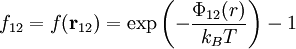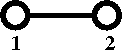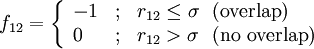# Difference between revisions of "Mayer f-function"

The Mayer f-function, or f-bond is defined as:$f_{12}=f({\mathbf r}_{12})= \exp\left(-\frac{\Phi_{12}(r)}{k_BT}\right) -1$

where

•$k_B$ is the Boltzmann constant.
•$T$ is the temperature.
•$\Phi_{12}(r)$ is the intermolecular pair potential.

Diagrammatically the Mayer f-function is written as## Hard sphere model

For the hard sphere model the Mayer f-function becomes:$f_{12}= \left\{ \begin{array}{lll} -1 & ; & r_{12} \leq \sigma ~~({\rm overlap})\\ 0 & ; & r_{12} > \sigma ~~({\rm no~overlap})\end{array} \right.$

where$\sigma$ is the hard sphere diameter.